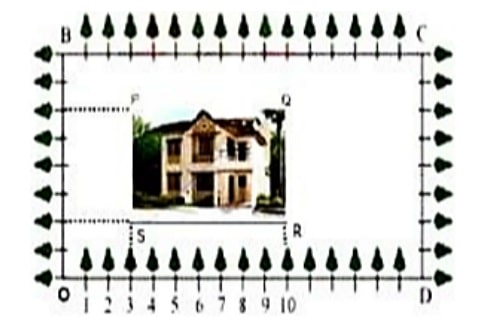Q) Using Cartesian Coordinates we mark a point on a graph by how far along and how far up it is.
The left-right (horizontal) direction is commonly called X-axis.
The up-down (vertical) direction is commonly called Y-axis.
In Green Park, New Delhi Suresh is having a rectangular plot ABCD as shown in the following figure. Sapling of Gulmohar is planted on the boundary at a distance of 1 m from each other. In the plot, Suresh builds his house in the rectangular area PQRS. In the remaining part of plot, Suresh wants to plant grass.i. Find the coordinates of the midpoints of the diagonal QS.
ii. Find the length and breadth of rectangle PQRS?
OR
Find the diagonal of rectangle.
iii. Find Area of rectangle PQRS.

Ans:

(i) coordinates of the midpoints of the diagonal QS:

Coordinates of Q = (10,6)

Coordinates of S = (3,2)

Midpoint of QS =(ii) Length and breadth of rectangle PQRS:

Coordinates of P = (3,6)

Coordinates of S = (3,2)

Breadth of PQRS = Distance between P and S == 4 m …. (i)

Coordinates of R = (10,2)

Coordinates of S = (3,2)

Length of PQRS = Distance between R and S == 7 m… (ii)

Therefore, Length of rectangle PQRS is 7 m and breadth is 4m.

OR

(ii) Find the diagonal of rectangle PQRS:

Coordinates of P = (3,6)

Coordinates of R = (10,2)

Diagonal of PQRS = Distance between P and R =m

Therefore, Diagonal of rectangle PQRS is √65 m.

(iii) Area of rectangle PQRS:

We know that the area of rectangle = length x breadth

from part (ii) above, we have calculated length = 7 m and breadth = 4m.

Therefore, area = 7 x 4 = 28 m2

Scroll to Top